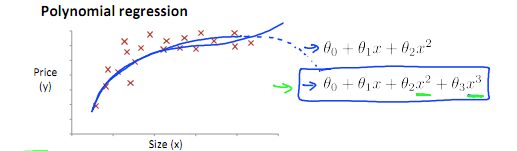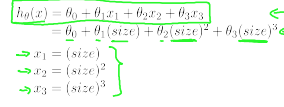# lsvih

0%

## 线性回归中的正则化（也可直接由$J(\theta )$求偏导得出。）

（在matlab中，下标号是从0开始，因此上式在matlab中表现是从$\theta _{2}$开始）

$\theta_{j} :=\theta_{j}(1-\alpha\frac{\lambda}{m})-\alpha\frac{1}{m}\sum^{m}_{i=1}(h_{\theta}(x^{(i)})-y^{(i)})x_{j}^{(i)}$（从第二项开始）

$1-\alpha \frac{\lambda}{m}$是小于1的

### 直线分类问题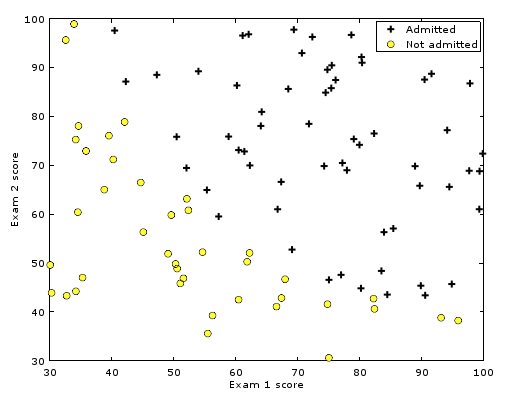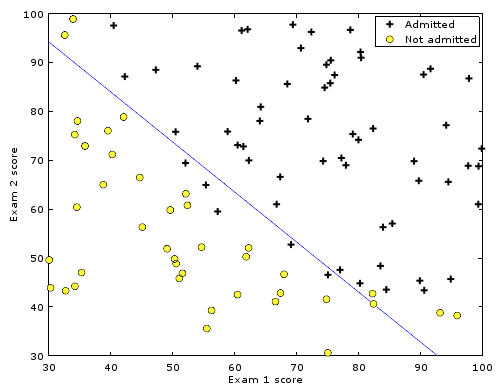### 非直线分类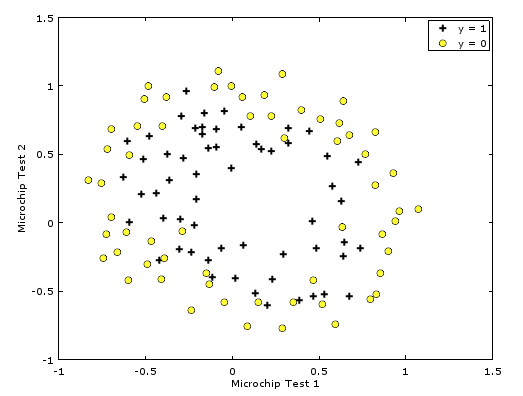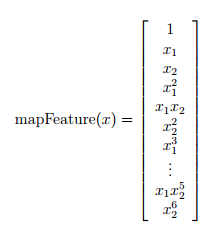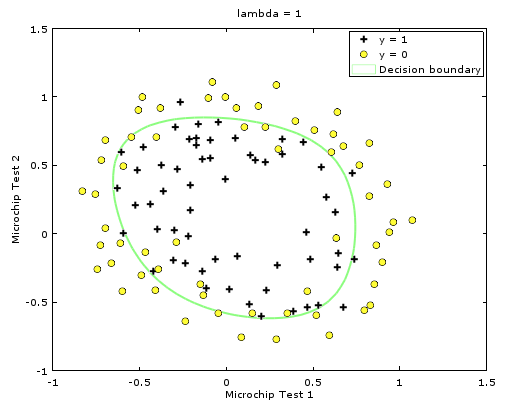$\lambda=0$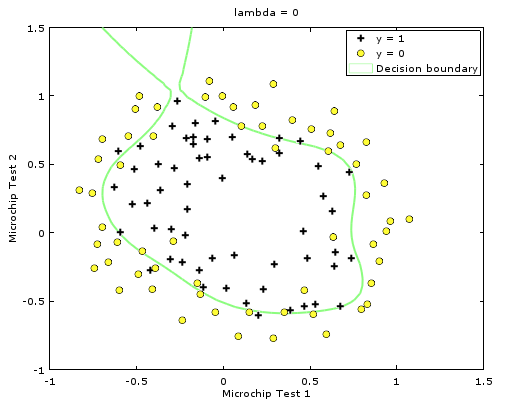$\lambda=100$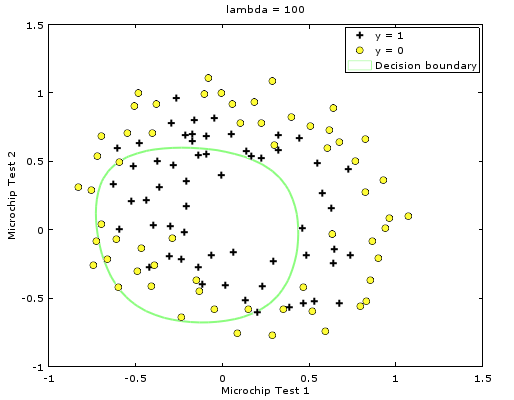y=0被称为负类，y=1被称为正类。通常上来说负类表示缺少某样东西(例如缺少而行肿瘤)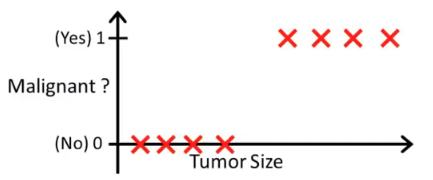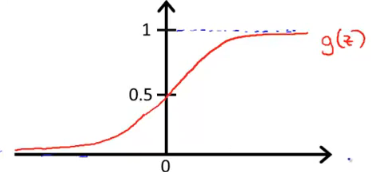g(z)在z趋近负无穷的时候趋近于0，z趋近正无穷的时候趋近与1.

### 概念：决策边界(decision boundary)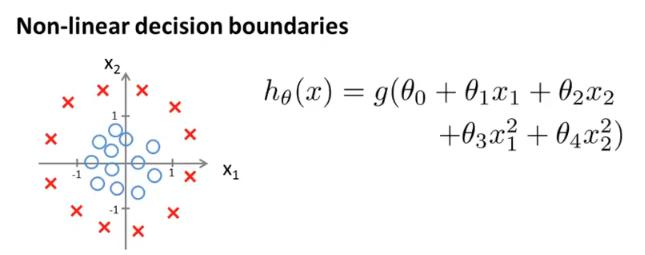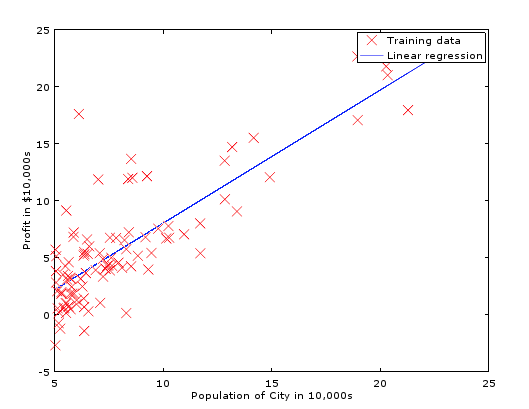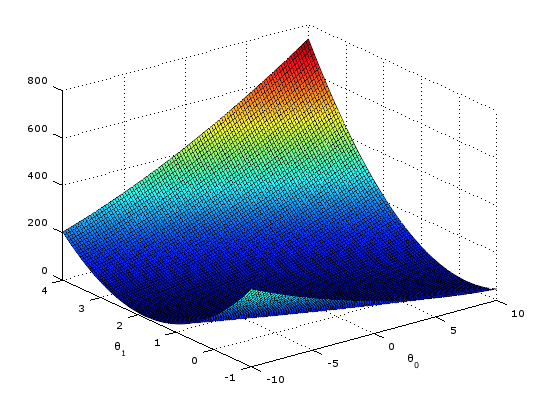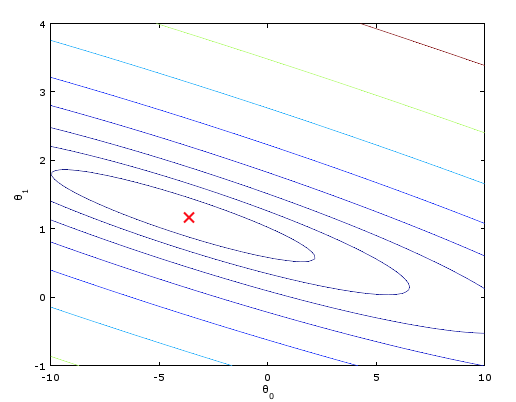indexOf之类的函数返回值为-1，可以通过取反符号将-1化为0，有利于编写判断式。

### 正规方程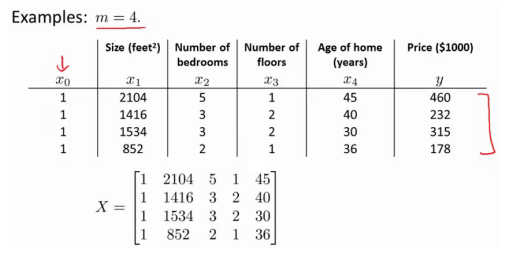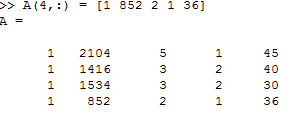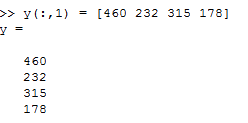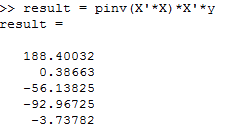## 特征缩放（Feature Scaling）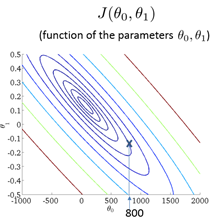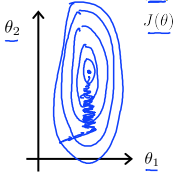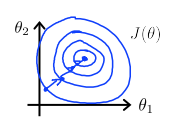### 多项式回归